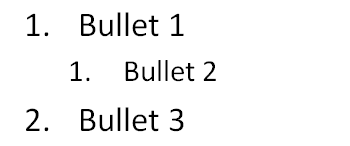# `<buAutoNum>` (Auto-Numbered Bullet)

This element specifies that automatic numbered bullet points should be applied to a paragraph. These are not just numbers used as bullet points but instead automatically assigned numbers that are based on both `<buAutoNum>` attributes and paragraph level.

example:```<p:txBody>
…
<a:p>
<a:pPr …>
<a:buAutoNum type="arabicPeriod"/>
</a:pPr>
…
<a:t>Bullet 1</a:t>
…
</a:p>
<a:p>
<a:pPr lvl="1"…>
<a:buAutoNum type="arabicPeriod"/>
</a:pPr>
…
<a:t>Bullet 2</a:t>
…
</a:p>
<a:p>
<a:pPr …>
<a:buAutoNum type="arabicPeriod"/>
</a:pPr>
…
<a:t>Bullet 3</a:t>
…
</a:p>
…
</p:txBody>```

For the above text there are a total of three bullet points. Two of which are at `<lvl="0">` and one at `<lvl="1">`. Due to this breakdown of levels, the numbering sequence that should be automatically applied will be 1, 1, 2 as is shown in the picture above. ]

Parent Elements

`<defPPr>`5.1.5.2.2); `<lvl1pPr>`5.1.5.4.13); `<lvl2pPr>`5.1.5.4.14); `<lvl3pPr>`5.1.5.4.15); `<lvl4pPr>`5.1.5.4.16); `<lvl5pPr>`5.1.5.4.17); `<lvl6pPr>`5.1.5.4.18); `<lvl7pPr>`5.1.5.4.19); `<lvl8pPr>`5.1.5.4.20); `<lvl9pPr>`5.1.5.4.21); `<pPr>`5.1.5.2.7)

Attributes

Description

`<startAt>` (Start Numbering At)

Specifies the number that will start number for a given sequence of automatically numbered bullets. When the numbering is alphabetical, the number should map to the appropriate letter. For instance 1 will map to 'a', 2 to 'b' and so on. If the numbers are larger than 26, then multiple letters should be used. For instance 27 should be represented as 'aa' and similarly 53 should be 'aaa'.

The possible values for this attribute are defined by the ST_TextBulletStartAtNum simple type (§5.1.12.63).

`<type>` (Bullet Autonumbering Type)

Specifies the numbering scheme that is to be used. This allows for the describing of formats other than strictly numbers. For instance a set of bullets may need to be represented by a series of Roman numerals instead of the standard 1,2,3,etc. number set.

The possible values for this attribute are defined by the ST_TextAutonumberScheme simple type (§5.1.12.61).

The following XML Schema fragment defines the contents of this element:

``````<complexType name="CT_TextAutonumberBullet">
<attribute name="type" type="ST_TextAutonumberScheme" use="required"/>
<attribute name="startAt" type="ST_TextBulletStartAtNum" use="optional" default="1"/>
</complexType>``````